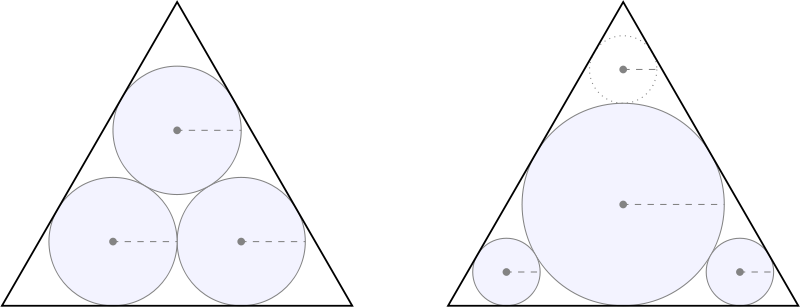# Malfatti CirclesWhat’s the best way to squeeze three circles into a triangle so that the area of the circles is maximized? In 1803 Italian mathematician Gian Francesco Malfatti decided that the best course was to place each circle tangent to the other two and to two sides of the triangle (left) — he thought that some instance of this arrangement would give the best solution.

But that’s not actually so: In an equilateral triangle, Malfatti’s circles occupy less area than the solution on the right, found by Lob and Richmond in 1930 — their suggestion is to inscribe the largest possible circle in the triangle, then fit the second circle into one of the triangle’s three corners, and then fit the third circle into one of the five spaces now available, taking the largest available option in each case.

In the case of an equilateral triangle, Lob and Richmond’s solution is only about 1% larger than Malfatti’s. But in 1946 Howard Eves pointed out that for a long, narrow isosceles triangle (below), simply stacking three circles can cover nearly twice the area of the Malfatti circles.

Subsequent studies have borne this out — it turns out that Malfatti’s plan is never best. We now know that Lob and Richmond’s procedure will always find three area-maximizing circles — but whether their approach will work for more than three circles is an open question.

(Thanks, Larry.)